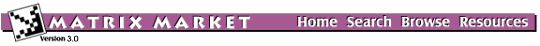## MVMODE: Model Eigenvalue Problem of ODEs

### from the NEP Collection

Matrix Generator MVMODE
Source: G.W. Stewart, University of Maryland
Discipline: Ordinary differential equations
Language: Fortran
Output format: matrix-vector multiply

Consider the following eigenvalue problem of an ordinary differential equation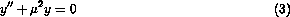with the boundary conditions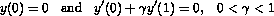.

It can be shown that the eigenvalues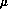are given by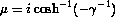,

which are complex. The solutions of this equation are of the form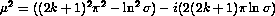for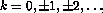, where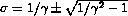.

The eigenproblem of (3) can be approximated by finite differences as follows. Let yi denote the approximate solution at the point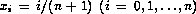. Replacing the second derivatives in (3) with a centered difference operators to obtain the generalized matrix eigenvalue problem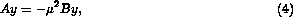,

for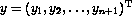, where with 1's on off-diagonals, -2 on diagonal, and an additional row appended with values (4,-1,... gamma, -4gamma, 3gamma)" >

and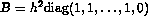. Problem (4) can be recast as the standard eigenvalue problem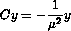,

where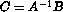.

The matrix-vector products Y = CX can be formed by solving the linear system AY = BX for Y using the banded Gaussian elimination. Fortran calling sequence for Y = CX.

In the data files,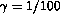.

Parameters:

 N the order of the matrix GAMMA boundary condition parameter

## Matrix Instances:

The Matrix Market is a service of the Mathematical and Computational Sciences Division / Information Technology Laboratory / National Institute of Standards and Technology.

[ Home ] [ Search ] [ Browse ] [ Resources ]

Last change in this page: Wed Sep 22 13:37:31 US/Eastern 2004 [Comments: ]